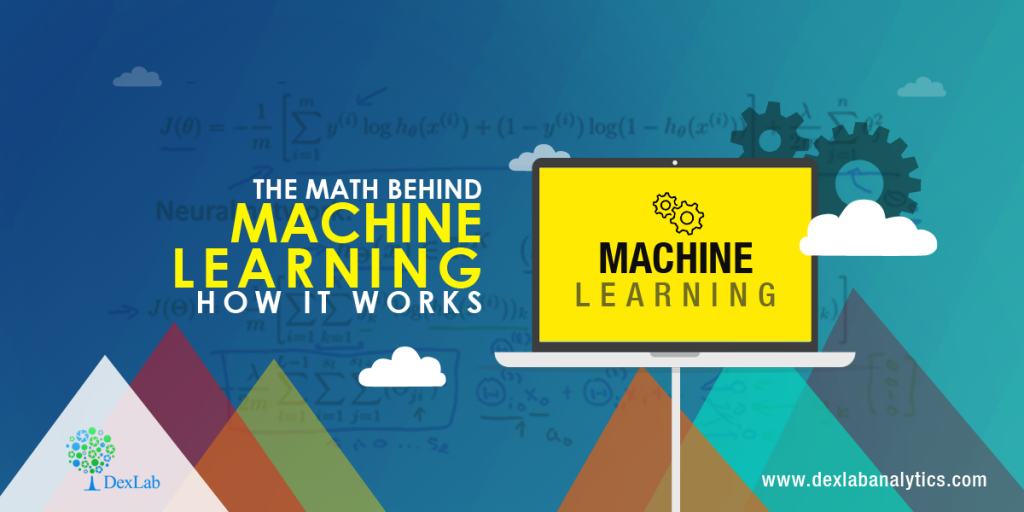# The Math Behind Machine Learning: How it Works

## Recent PostsIt is evident that in the last few months, we have had several people showcase their enthusiasm about venturing into the world of data science using Machine Learning techniques. They are keen on probing the statistical regularities and building impeccable data-driven products. but we have made an observation that some may actually lack the necessary mathematical knowledge and intuition to get the framework for achieving results with data. And this is why we have decided to discuss this lacking through our blog.

In the recent times, there has been a noticeable upsurge in the availability of several easy-to-use machine and deep learning packages such as Weka, Tensorflow, scikit learn etc. But you must understand that machine learning as a field is one that has both statistical concepts, probabilistic concepts, computer science and algorithmic concepts to arise from learning intuitively from available data and also is about determining the patterns and hidden insights, which can be used to build intelligent applications. While still having the immense possibilities of Machine Learning and Deep Learning which is a thorough mathematical understanding of many of these techniques which is necessary for a good grasp of the internal workings of algorithms to achieve a good result.

Enrol in the most comprehensive machine learning course in India with us.

#### Why we must think about the math?

To explain why it is necessary to behind the scenes into the mathematical details of Machine Learning, we have put own a few important points:

1. To choose the right algorithm which will include giving considerations, to accuracy, to the right training time, complexity of model, number of parameters and the number of features.
2. To choose parameter settings and to validate the strategies
3. To indentify the under-fitting and over-fitting by understanding the bias-variance trade off.
4. For acquiring ample confidence about the interval and uncertainty

#### The level of math one will need:

The primary question when one tries to understand an interdisciplinary field such as Machine Learning, is the amount of math needed and the level of math needed to understand these techniques.

The answer to this question is not as simple as it may seem and is multidimensional which, depends upon the level and interest of the individual. Research conducted in these mathematical formulations and theoretical advancements for Machine Learning is an ongoing process and a few researchers are already working on few more advanced techniques. However, we will state the least amount of math that is a must have skill for being a successful Machine learning Engineer/ Scientist is the importance of each and every mathematical concept.

#### Linear algebra:

This is the math skill to have for the 21st century. One must be well-versed with the topics of Principal Component Analysis (PCA), Singular Value Decomposition (SVD), Eigendecomposition of a matrix, LU Decomposition, QR Decomposition/Factorization, Symmetric Matrices, Orthogonalization & Orthonormalization, Matrix Operations, Projections, Eigenvalues & Eigenvectors, Vector Spaces as these norms are absolutely necessary for the understanding and the optimization methods for machine learning. The best thing about linear algebra is that there are a lot of online resources.

#### Probability theory and statistics:

Machine learning and statistics are not too different a field. And in reality some people have actually defined Machine Learning as “doing statistics on a Mac”. A few fundamentals that are a must have for machine learning are – Combinatorics, Probability Rules & Axioms, Bayes’ Theorem, Random Variables, Variance and Expectation, Conditional and Joint Distributions, Standard Distributions (Bernoulli, Binomial, Multinomial, Uniform and Gaussian), Moment Generating Functions, Maximum Likelihood Estimation (MLE), Prior and Posterior, Maximum a Posteriori Estimation (MAP) and Sampling Methods.

#### Multivariate calculus:

Differential and Integral Calculus, Partial Derivatives, Vector-Values Functions, Directional Gradient, Hessian, Jacobian, Laplacian and Lagragian Distribution are some of the necessary topics necessary for understanding ML.#### Algorithms and Complex Optimizations:

In order to realize the computational efficiency and scalability of our Machine Learning Algorithm and for exploiting the sparsity in the dataset, this concept is necessary. One must have knowledge of data structures such as Binary Trees, Hashing, Heap, Stack etc, and Dynamic Programming, Randomized & Sublinear Algorithm, Graphs, Gradient/Stochastic Descents and Primal-Dual methods.

A few other mathematical skills that are often necessary for understanding ML are the following Real and Complex Analysis (Sets and Sequences, Topology, Metric Spaces, Single-Valued and Continuous Functions, Limits), Information Theory (Entropy, Information Gain), Function Spaces and Manifolds.

Machine learning training in Gurgaon from experts with in-depth instruction on math skills is offered at DexLab Analytics. Check out our Machine learning certification brochure for the same at the website.

.### Call us to know more### Gurgaon+91 931 572 5902